### Partial Differential Equations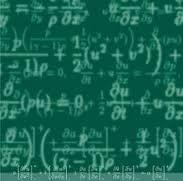The objective of this course is to understand basic methods for solving Partial Differential Equations of first order and higher order.

### Probability & Statistical Inference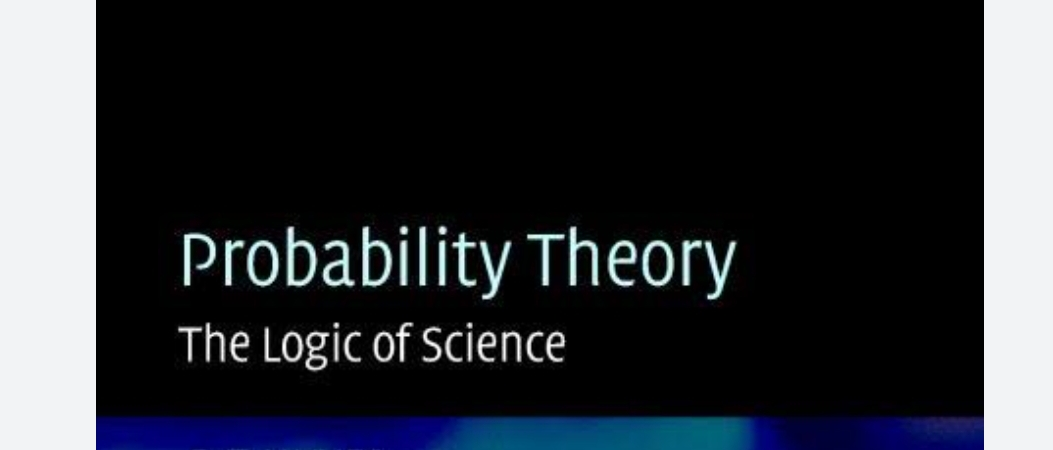The probability and statistical inference course has great application in engineering technology, biological sciences and many more problems in real life situations.  The knowledge on this course will provide the basis for further studies in advanced course like Stochastic process, Statistical Methods etc. which forms the basics of higher mathematics.

### Ordinary differential equations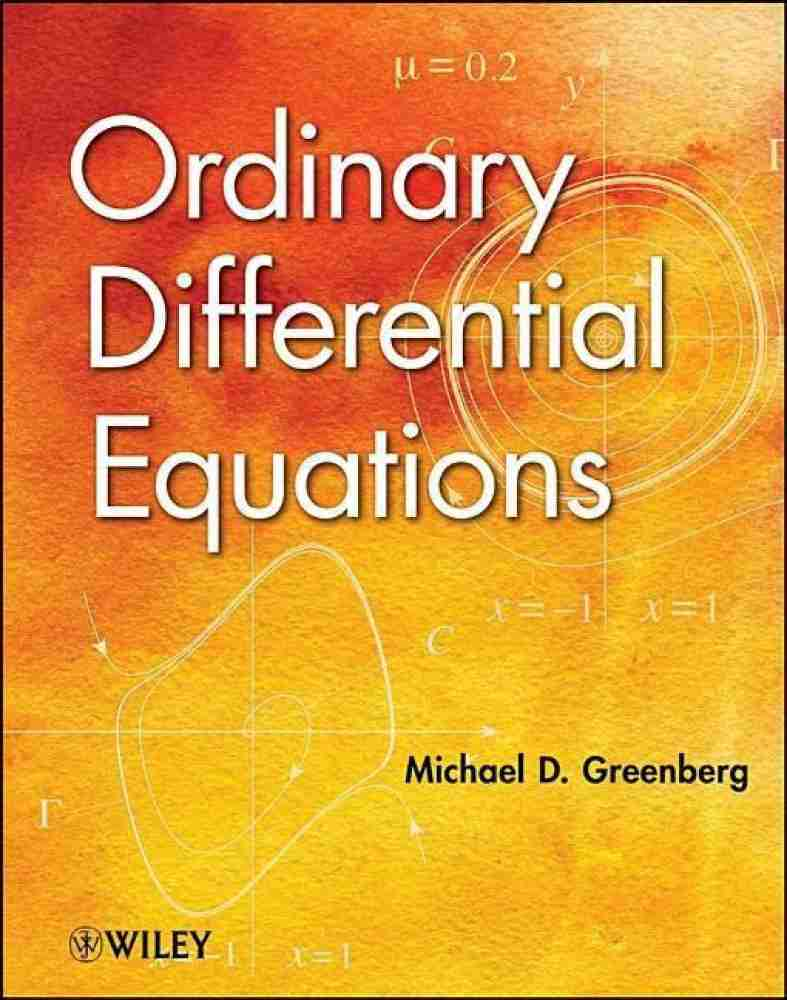Differential Equations introduced by Leibnitz in 1676 models almost all physical, biological, Chemical, Socio-economic system in nature. The objective of this course is to familiarize the students with various methods of solving differential equations and to have a qualitative analysis of the behaviour of solutions along with existence and uniqueness problems.

### Complex Analysis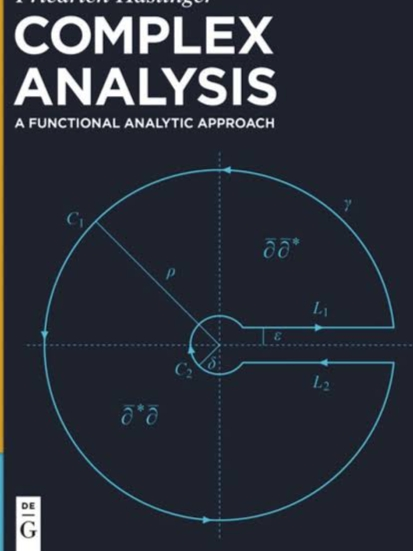•  Deals with the study of complex Number

•  Analytic Function

• Taylor's Series

### Real Analysis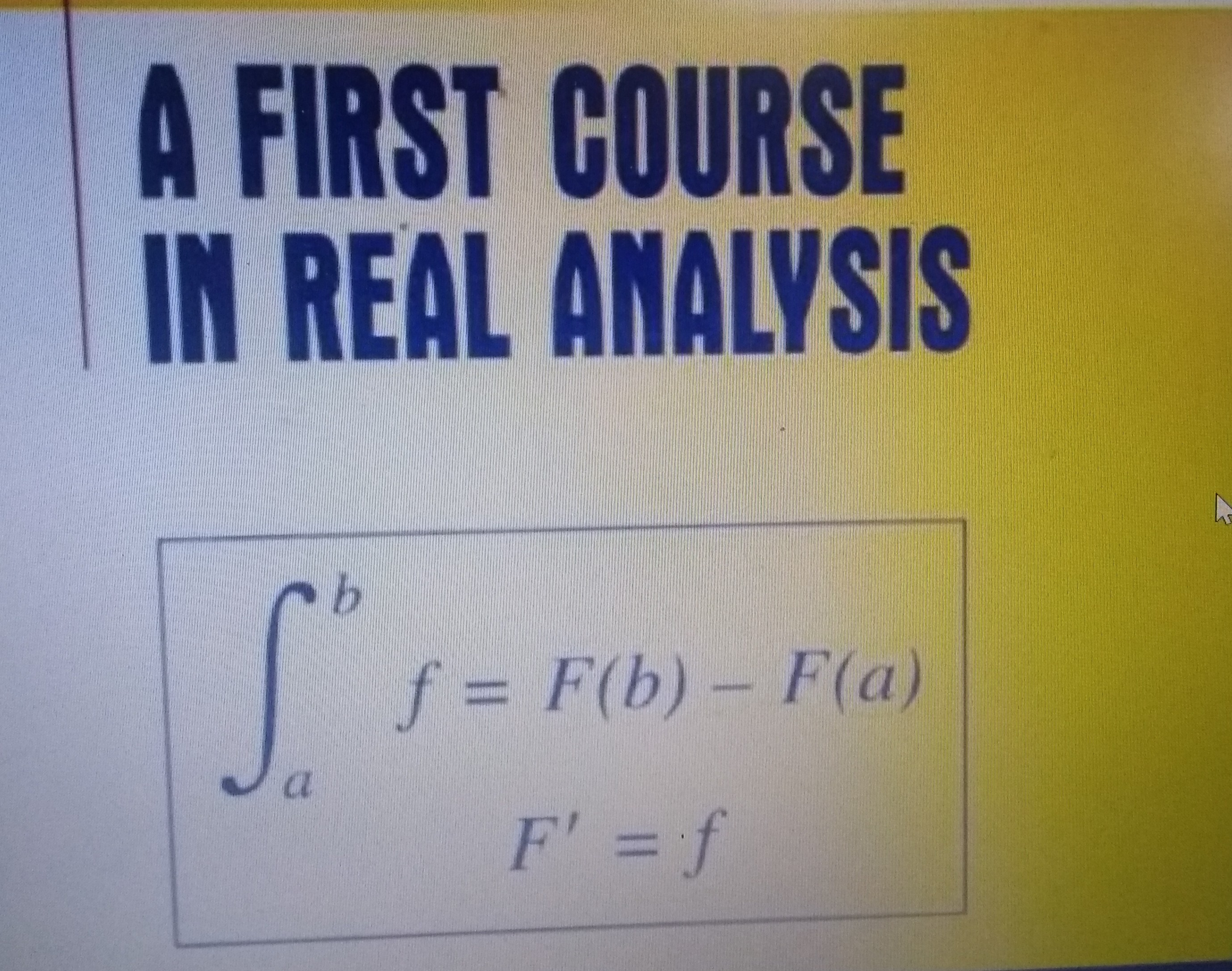The objective of this course is to give basic materials to students so that they can easily understand the structure and topology of real number system, sequences and series of real numbers and functions, Riemann Integration.

 After completing this course students can make a choice for further studies in Complex Analysis, Functional Analysis, Measure Theory, Differential Geometry, Advanced Analysis, Harmonic Analysis etc.